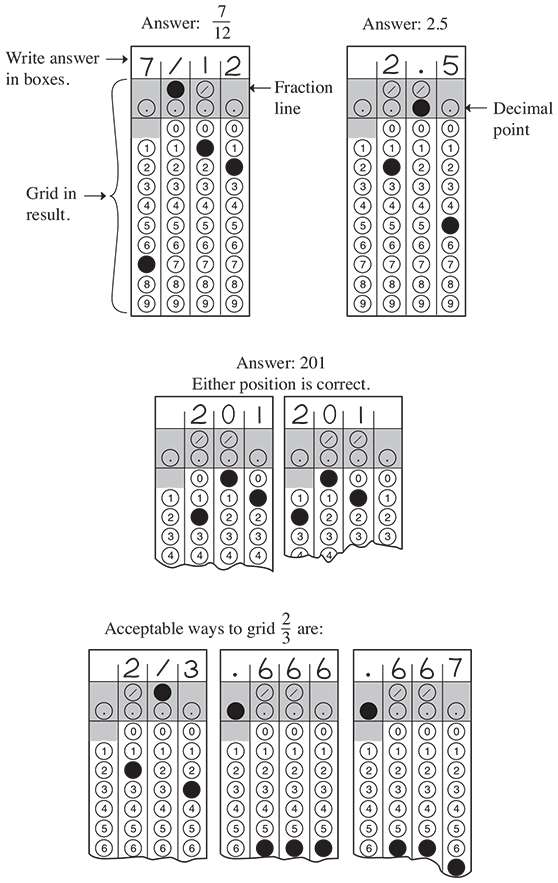# SAT - Math Test Summary

Working...Math Test - Summary

The SAT Math Test covers a range of math practices, with an emphasis on problem solving, modeling, using tools strategically, and using algebraic structure.

Instead of testing you on every math topic there is, the new SAT asks you to use the math that you’ll rely on most in all sorts of situations. Questions on the Math Test are designed to mirror the problem solving and modeling you’ll do in:

• College math, science, and social science courses

• The jobs that you hold

For instance, to answer some questions you’ll need to use several steps —because in the real world a single calculation is rarely enough to get the job done.

Quick Facts:

• Most math questions will be multiple choice, but some — called grid-ins — ask you to come up with the answer rather than select the answer.

• The Math Test is divided into two portions: Math Test – Calculator and Math Test – No Calculator.

• Some parts of the test include several questions about a single scenario.

Focus:

The Math Test will focus in depth on the three areas of math that play the biggest role in a wide range of college majors and careers:

The Math Test also draws on Additional Topics in Math, including the geometry and trigonometry most relevant to college and career readiness.

What the Math Test Measures:

 Fluency Conceptual Understanding Applications The Math Test is a chance to show that you: Carry out procedures flexibly, accurately, efficiently, and strategically. Solve problems quickly by identifying and using the most efficient solution approaches. This might involve solving a problem by inspection, finding a shortcut, or reorganizing the information you’ve been given. You’ll demonstrate your grasp of math concepts, operations, and relations. For instance, you might be asked to make connections between properties of linear equations, their graphs, and the contexts they represent. These real-world problems ask you to analyze a situation, determine the essential elements required to solve the problem, represent the problem mathematically, and carry out a solution.

Calculator Use:

Calculators are important tools, and to succeed after high school, you’ll need to know how — and when — to use them. In the Math Test – Calculator portion of the test, you’ll be able to focus on complex modeling and reasoning because your calculator can save you time.

However, the calculator is, like any tool, only as smart as the person using it. The Math Test includes some questions where it’s better not to use a calculator, even though you’re allowed to. In these cases, students who make use of structure or their ability to reason will probably finish before students who use a calculator.

The Math Test – No Calculator portion of the test makes it easier to assess your fluency in math and your understanding of some math concepts. It also tests well-learned technique and number sense.

Grid-In Questions:

Although most of the questions on the Math Test are multiple choice, 22 percent are student-produced response questions, also known as grid-ins. Instead of choosing a correct answer from a list of options, you’ll need to solve problems and enter your answers in the grids provided on the answer sheet.

• Mark no more than one circle in any column.

• Only answers indicated by filling in the circle will be scored (you won’t receive credit for anything written in the boxes located above the circles).

• It doesn't matter in which column you begin entering their answers; as long as the responses are recorded within the grid area, you’ll receive credit.

• The grid can hold only four decimal places and can only accommodate positive numbers and zero.

• Unless a problem indicates otherwise, answers can be entered on the grid as a decimal or a fraction.

• Fractions like “3/24” do not need to be reduced to their lowest terms.

• All mixed numbers need to be converted to improper fractions before being recorded in the grid.

• If the answer is a repeating decimal, students must grid the most accurate value the grid will accommodate.

Below is a sample of the instructions students will see on the test.